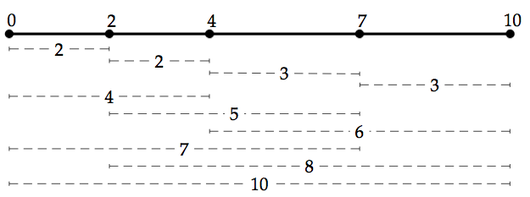# Glossary

## Difference multiset

The difference multiset $\Delta X$ corresponding to a set $X$ of numbers is the multiset containing the positive differences of pairs of elements of $X$. Accordingly, $\Delta X$ is a subset of the Minkowski difference $X \ominus X$, which contains both positive and negative differences of pairs of elements of $X$.

We can visualize the difference multiset by placing the elements of $X$ along an interval of the real line. For example, consider the set $X = \{0, 2, 4, 7, 10\}$, whose elements are assigned to the interval in the figure below. By measuring the distance between any pair of points, shown by dotted lines, we can see that $\Delta X$ must be $\{2, 2, 3, 3, 4, 5, 6, 7, 8, 10\}$.Note that the difference multiset contains one element for every pair of elements of $X$, so that if $X$ contains $n$ elements, $\Delta X$ must contain $\binom{n}{2}$ elements (see combination statistic).